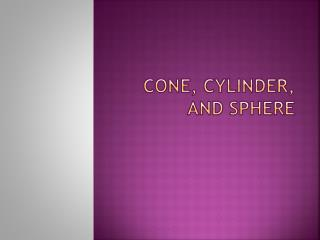DownloadDownload PresentationCone, Cylinder, and Sphere

# Cone, Cylinder, and Sphere

Download Presentation## Cone, Cylinder, and Sphere

- - - - - - - - - - - - - - - - - - - - - - - - - - - E N D - - - - - - - - - - - - - - - - - - - - - - - - - - -
##### Presentation Transcript

1. Cone, Cylinder, and Sphere

2. Element of Cone A cone has two parts, namely the base and the lateral. his the height of the cone, r is the radius of the base, and s is the slant height. Lateral s h Base r Lateral Base s r

3. Element of Cylinder Flat Top Flat Top h Lateral Lateral r Flat Base Flat Base A cylinder has three parts, namely the flat top, flat base and the lateral. h is the height of the cylinder and r is the radius of the base.

4. Element of Sphere r is radius of sphere and p is point centre. r p

5. Nets of Cone

6. Nets of Cylinder

7. Surface area of Cylinder • Look at the net of the cylinder below. The total surface area can be found by adding all the three areas.

8. Total surface area = Lateral area + (2Xbase area)

9. Surface area of Cone • A cone has two parts, namely the base and the lateral. • On Figure, t is the height of the cone, r is the radius of the base, and s is the slant height.

10. If the cone is cut along the slant s and its base, we will get the cone net which consists of sector which has radius s and a circle with radius r, as shown:

11. Surface area of Sphere • How do you find the surface area of a sphere? • The surface area of sphere same with the lateral of Cylinder. Surface area of Sphere = Lateral area of cylinder = 2πrh = 2πr X 2r S = 4πr₂

12. Exercise • Determine the total surface area of this cylinder.

13. Find the volume and the total surface area of each of the following cones. (π = 3.14)

14. Volume ofCone

15. Find the formula Do you remember about the formula of pyramid? Volumeof pyramid = 1/3 x Area of Base x Height Because the cone base is a circle with radius r, then Area of Base= π r 2

16. Find the formula Actually the cone is a pyramid, because have top point. (titik puncak) and altitude. Butthe cone’sbase is a circle with radius r, So theArea of Circle = π r 2 (base of cone)

17. Find the formula From this process so the volume of cone is same as volume of pyramid. Volume of cone = 1/3 Area of Base x Height = 1/3 x π r 2 x h Therefore the Volume of Cone =1/3 π r 2 h

18. Volume ofCylinder

19. Volume of Cylinder Let’s we see this pictures Can you compare them ? Actually the cylinder is a prism, because the base and top side are parallel and congruent. But the cylinder have base a circle So the volume of prism = volume of cylinder

20. Area of Base x Height Volume of Prism= Area of Base x Height Volume of Cylinder= Because the cylinder’s base is a circle so the base area is r.r r.r x h = = π r 2 x h Hence the volume of cylinder = π r 2 x h

21. Find the Volume of Sphere Prepare the instrument and materials : Scissor, Cutter, Rice, Plastic ball The ways........... • Cut the ball become 2 parts 2. Make a coneby theheightand the radius same with radius of ball 3. Fill the cone by rice until full flat. And pour to the one of hemisphere 4. Repeat until all of hemisphere is full flat. And note it.

22. Volume of Sphere Height of cone = radius of sphere= r

23. Volume of Sphere From theis activity we see that volume of rice that poured to hemisphere is not change. It means that the volume of hemisphere = 2 times of cone Volume of cone = 1/3 π r 2 h (h=r) = 1/3 π r 2 r Volume of hemisphere = 2 x 1/3 π r 2 r so Volume of Sphere = 4 x 1/3 π r 2 r = 4/3 π r 3

24. Design The Cone Picture Make an ellipse Find and mark the centre point of ellipse Make an altitude Mark this end point of altitude by O. Connect the O lie ellipse o r

25. Design The Cylinder Picture Make an ellipse Make parallel line that lie side of ellipse Make ellipse once again to upward.

26. Design The Sphere Picture Make an circle Find and mark the centre point of circle. Make ellipse from centre point of circle